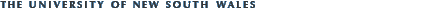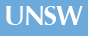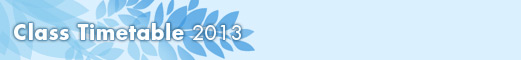Class search by Subject Area

 Data is correct as at Wed, 11-09-2013 01:37:28 EST Go To New search by Campus/Subject Area Data is currently published for 2013 and is subject to change. New search by Teaching Period

 Campus Sydney Subject Area Mathematics

Subject Area Listing
 Code Course Title Units of Credit MATH1011 Fundamentals of Mathematics B 6 MATH1031 Mathematics for Life Sciences 6 MATH1041 Statistics for Life and Social Sciences 6 MATH1081 Discrete Mathematics 6 MATH1131 Mathematics 1A 6 MATH1141 Higher Mathematics 1A 6 MATH1151 Mathematics for Actuarial Studies and Finance 1A 6 MATH1231 Mathematics 1B 6 MATH1241 Higher Mathematics 1B 6 MATH1251 Mathematics for Actuarial Studies and Finance 1B 6 MATH2011 Several Variable Calculus 6 MATH2019 Engineering Mathematics 2E 6 MATH2069 Mathematics 2A 6 MATH2089 Numerical Methods and Statistics 6 MATH2099 Mathematics 2B 6 MATH2111 Higher Several Variable Calculus 6 MATH2120 Mathematical Methods for Differential Equations 3 MATH2130 Higher Mathematical Methods for Differential Equations 3 MATH2241 Introduction to Atmosphere and Ocean Dynamics 6 MATH2301 Mathematical Computing 6 MATH2400 Finite Mathematics 3 MATH2501 Linear Algebra 6 MATH2520 Complex Analysis 3 MATH2601 Higher Linear Algebra 6 MATH2620 Higher Complex Analysis 3 MATH2701 Abstract Algebra and Fundamental Analysis 6 MATH2801 Theory of Statistics 6 MATH2831 Linear Models 6 MATH2859 Probability, Statistics and Information 3 MATH2871 Data Management for Statistical Analysis 6 MATH2881 Quantitative Risk 6 MATH2901 Higher Theory of Statistics 6 MATH2931 Higher Linear Models 6 MATH3000 Mathematics/Statistics Project 3 MATH3001 Mathematics/Statistics Project 6 MATH3002 Mathematics/Statistics Project 12 MATH3041 Mathematical Modelling for Real World Systems 6 MATH3101 Computational Mathematics 6 MATH3121 Mathematical Methods and Partial Differential Equations 6 MATH3161 Optimisation 6 MATH3201 Dynamical Systems and Chaos 6 MATH3261 Fluids, Oceans and Climate 6 MATH3311 Mathematical Computing for Finance 6 MATH3411 Information, Codes and Ciphers 6 MATH3511 Transformations, Groups and Geometry 6 MATH3521 Algebraic Techniques in Number Theory 6 MATH3560 History of Mathematics 3 MATH3570 Foundations of Calculus 3 MATH3611 Higher Analysis 6 MATH3701 Higher Topology and Differential Geometry 6 MATH3711 Higher Algebra 6 MATH3801 Probability and Stochastic Processes 6 MATH3811 Statistical Inference 6 MATH3821 Statistical Modelling and Computing 6 MATH3831 Statistical Methods in Social and Market Research 6 MATH3841 Statistical Analysis of Dependent Data 6 MATH3851 Experimental Design and Categorical Data 6 MATH3901 Higher Probability and Stochastic Processes 6 MATH3911 Higher Statistical Inference 6 MATH4011 Quantitative Risk Thesis / Project A 6 MATH4012 Quantitative Risk Thesis / Project B 12 MATH4103 Applied Mathematics Honours (Full Time) 24 MATH4104 Applied Mathematics Honours (Part Time) 12 MATH4603 Pure Mathematics Honours (Full Time) 24 MATH4604 Pure Mathematics Honours (Part Time) 12 MATH4903 Statistics Honours (Full Time) 24 MATH4904 Statistics Honours (Part Time) 12 MATH6781 Biomathematics 6